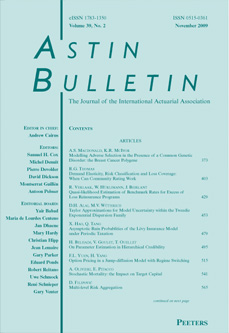this issueprevious article in this issue next article in this issueDocument Details : Title: A Frequency Distribution Method for Valuing Average Options Author(s): NEAVE, E. Journal: ASTIN Bulletin Volume: 27    Issue: 2   Date: November 1997   Pages: 173-205 DOI: 10.2143/AST.27.2.542047 Abstract : This paper finds payoff frequency distributions for valuing European and American fixed strike average options on a discrete time, recombining multiplicative binomial asset price process. In comparison to other discrete valuation methods the distributions, obtained analytically from a generating function, greatly reduce the computational requirements needed for accurate valuation. Less data are needed to value geometric than arithmetic averages, but the magnitude of calculations is similar for both instruments. Calculations of order T³ are needed to value European instruments, of order T 4 to value their American counterparts. A frequency distribution of a quantity called path sums is used to value geometric average options, and a joint distribution of path sums and realized prices is used to value arithmetic average options. The frequency distributions give an exact value for geometric average, an approximate value for arithmetic average instruments. The method obtains additional information from the generating function to estimate approximation errors relative to the exact binomial solution. If the errors are significant they can be reduced using still further detail from the generating function. Error reduction can be performed selectively to minimize additional calculation.

34.207.247.69.